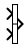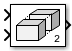Documentation

# Vector Concatenate, Matrix Concatenate

Concatenate input signals of same data type to create contiguous output signal

• Library:
• Simulink / Commonly Used Blocks

Simulink / Math Operations

Simulink / Signal Routing

HDL Coder / Math Operations

HDL Coder / Signal Routing## Description

The Concatenate block concatenates the input signals to create an output signal whose elements reside in contiguous locations in memory.

### Tip

The Concatenate block is useful for creating an output signal that is nonvirtual. However, to create a vector of function calls, use a Mux block instead.

You use a Concatenate block to define an array of buses. For details about defining an array of buses, see Combine Buses into an Array of Buses.

The Concatenate block operates in either vector or multidimensional array concatenation mode, depending on the setting of its Mode parameter. In either case, the block concatenates the inputs from the top to bottom, or left to right, input ports.

### Vector Mode

In vector mode, all input signals must be either vectors or row vectors (1-by-M matrices) or column vectors (M-by-1 matrices) or a combination of vectors and either row or column vectors. When all inputs are vectors, the output is a vector.

If any of the inputs are row or column vectors, the output is a row or column vector, respectively.

### Multidimensional Array Mode

Multidimensional array mode accepts vectors and arrays of any size. It assumes that the trailing dimensions are all ones for input signals with lower dimensionality. For example, if the output is 4-D and the input is `[2x3]` (2-D), this block treats the input as `[2x3x1x1]`. The output is always an array. The Concatenate dimension parameter allows you to specify the output dimension along which the block concatenates its input arrays.

If you set the Concatenate dimension parameter to `2` and inputs are 2-D matrices, the block performs horizontal matrix concatenation and places the input matrices side-by-side to create the output matrix. For example, see the ex_concatenate_horizontal model:If you set the Concatenate dimension parameter to `1` and inputs are 2-D matrices, the block performs vertical matrix concatenation and stacks the input matrices on top of each other to create the output matrix. For example, see the ex_concatenate_vertical model:For horizontal concatenation, the input matrices must have the same column dimension. For vertical concatenation, the input matrices must have the same row dimension. All input signals must have the same dimension for all dimensions other than the concatenation dimensions.

If you set the Mode parameter to ```Multidimensional array```, the Concatenate dimension parameter to `3`, and the inputs are 2-D matrices, the block performs multidimensional matrix concatenation. For example, see the ex_concatenate_multidims model:## Ports

### Input

expand all

First input to concatenate, specified as a scalar, vector, matrix, or N-D array.

#### Dependencies

• Inputs must be of the same data type.

• Matrix and N-D array inputs are supported only when you set Mode to ```Multidimensional array```.

Data Types: `single` | `double` | `int8` | `int16` | `int32` | `uint8` | `uint16` | `uint32` | `Boolean` | `fixed point` | `enumerated` | `bus`

Nth input to concatenate, specified as a scalar, vector, matrix, or N-D array.

#### Dependencies

• To enable this port, set Number of inputs to an integer greater than or equal to 2.

• Inputs must be of the same data type.

• Matrix and N-D array inputs are supported only when you set Mode to ```Multidimensional array```.

Data Types: `single` | `double` | `int8` | `int16` | `int32` | `uint8` | `uint16` | `uint32` | `Boolean` | `fixed point` | `enumerated` | `bus`

### Output

expand all

Concatenation of input signals, along specified dimension. Outputs have the same data type as the input.

Data Types: `single` | `double` | `int8` | `int16` | `int32` | `uint8` | `uint16` | `uint32` | `Boolean` | `fixed point` | `enumerated`

## Parameters

expand all

Specify the number of inputs for the block as a real-valued, positive integer, less than or equal to 65536.

#### Programmatic Use

 Block Parameter: `NumInputs` Type: character vector Values: positive integer Default: `'2'`

Select the type of concatenation that this block performs. The default Mode of the Vector Concatenate block is `Vector`. The default Mode of the Matrix Concatenate block is ```Multidimensional array```.

• When you select `Vector` the block performs vector concatenation (see Vector Mode for details).

• When you select `Multidimensional array`, the block performs matrix concatenation (see Multidimensional Array Mode for details).

#### Programmatic Use

 Block Parameter: `Mode` Type: character vector Values: `'Vector' | 'Multidimensional array'` Default: `'Vector'`

Specify the output dimension along which to concatenate the input arrays.

• To concatenate input arrays vertically, enter `1`.

• To concatenate input arrays horizontally, enter `2`.

• To perform multidimensional concatenation on the inputs, specify an integer greater than 2.

#### Dependencies

To enable this parameter, set Mode to `Multidimensional array`.

#### Programmatic Use

 Block Parameter: `ConcatenateDimension` Type: character vector Values: scalar integer Default: `'1'`

## Block Characteristics

 Data Types `Boolean` | `double` | `enumerated` | `fixed point` | `integer` | `single` Direct Feedthrough `yes` Multidimensional Signals `no` Variable-Size Signals `yes` Zero-Crossing Detection `no`